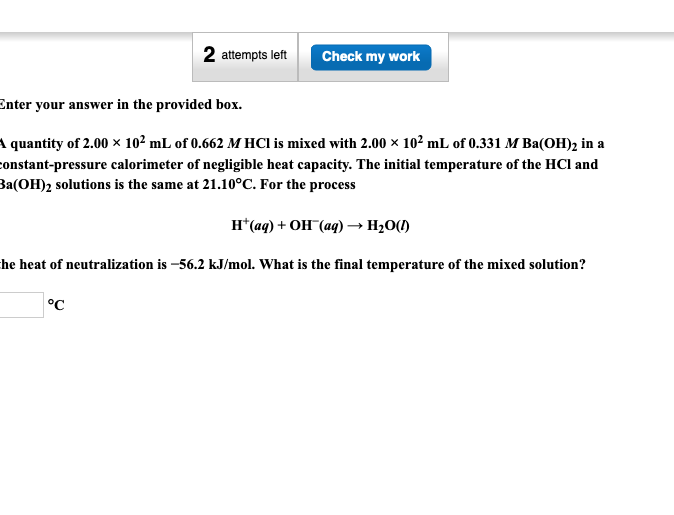# A quantity of 2.00 ×102 mL of 0.662 M HCl is mixed with 2.00 ×102 mL of 0.331 M Ba(OH)2 in a constant-pressure calorimeter of negligible heat capacity. the initial temperature of the HCl and Ba(OH)2 solution is the same at 21.10°C. For the process H+(aq) + OH-(aq) -> H2O(l), the heat of neutralization is -56.2 kJ/mol. What is the final temperature of the mixed solution?